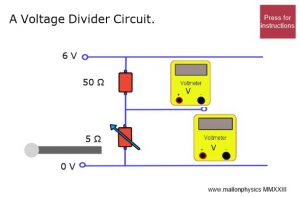# Charge that capacitor!⤴

from @ HelpmyphysicsA capacitor can be used in a sensor circuit to switch on a transistor after a time interval.
Such a voltage divider circuit will act as a kind of timer.
The time the voltage across the capacitor reaches 0.7 v, to switch on the transistor, can be short or long depending on the size of the capacitance of the capacitor or the size of the resistor its being charged through.

To lengthen the time interval the resistance can be made bigger or the vakue of the capacitor’s capacitance can be made bigger.

# Share the voltage!⤴

from @ HelpmyphysicsClick the picture to go to the sim.

Some questions:

1. What do you notice about the variable resistor’s voltage as its resistance increases?
2. What do you notice about the sum of the voltages across the resistors?
3. For each resistor in turn, take the ratio of voltage across it and divide by the resistance. What do you notice?

# More on coding the normal force.⤴

from @ Helpmyphysics

In this code I have added a slider to change the value of the acceleration of the floor.
Think of the floor of an elevator.

A positive acceleration indicates that the elevator is accelerating upwards and a negative acceleration indicates a downward acceleration.

Press the play button to start the program and then the pencil button to examine the code

# Coding the Doppler effect⤴

from @ Helpmyphysics

The black car is moving towards an observer,represented by the green ball, while sounding its horn.
The sound waves spread out from the car. Notice that the sound waves squash up as the car approaches the observer and stretch out as the car leaves the observer behind.
Press the run button to start the program.
Can you see why the observer hears a higher frequency of sound as the car approaches and a lower frequency of sound as the car moves away?